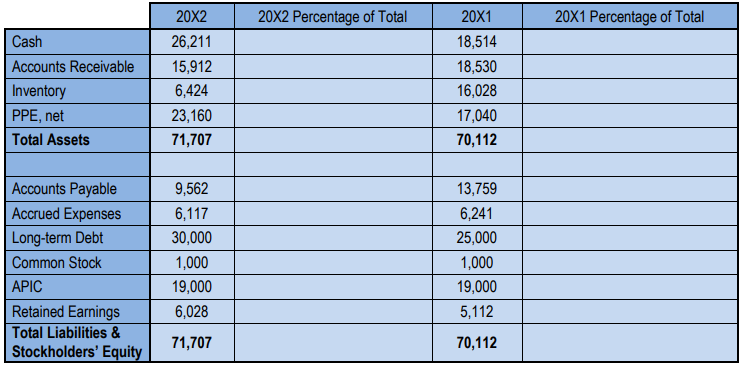## Financial Accounting

Learn the toughest concepts covered in your Financial Accounting class with step-by-step video tutorials and practice problems.

14. Financial Statement Analysis

# Vertical Analysis

1
concept

## Vertical Analysis8m
Play a video:
2
example

## Vertical Analysis4m
Play a video:
Alright, so let's run through 2017 and do our income statement vertical analysis. So notice this time we're still dealing with the same base our bases still net sales, but we've got to use the 2017 number. Right? Now we're gonna use 58 oh 81 as our denominator. Okay. Before we're using 65455 as a denominator because it was 2018. Well, for 2017 we use their net sales number and it's gonna be the same thing. Right? We're going to see that for Sorry for net sales. Well, it's always gonna be 100% right? It's always 100% of itself because it is our base. Cool. So let's start going down. I'll write a couple of these out and then we'll see that it's very much the same as what we did 45377. Right, This is our cost of goods sold, divided by 58 oh 81 And that gives us 78.1 right, 78.1%. Uh once we multiply by 100 there we do the same thing with gross profit. 12 704 divided by 58 oh 81 right, Same base amount all the way down. And it's gonna be 21.9%. And this should make sense. Right? If we have 100% in net sales and 78.1 went to pay for the cost of goods sold, well, whatever leftover is gross profit. So the 100% minus the 78.1 should be 21.9 and it is all right, So that should make sense, right? We kept the 21.9 and this is where it starts to be beneficial to. Not only can we make that analysis going down, but now we can compare year by year two, we can kind of do some sort of horizontal analysis with our vertical analysis where we see that we had 16.1% as our gross profit in 2018, 21.9% in 2017. So that's gone down a bit. That could be important information to an investor to a bank that's loaning us money, right? They would like to know this information. Even the company itself gets good information out of this. So let's keep going down here. The next one we'll rent, expense. It didn't change year over year 2411, but It's coming out of less net sales, right? So, we can imagine it's gonna be a bigger percentage because we've got a smaller denominator. So that's gonna be a bigger percentage of it. So, right now it's 4.2%. Once we do that and multiplied by 100 we get 4.2%. Cool. So, you can see the math is very much the same as what we did in 2018. I'm gonna start going down a little faster here. Let me get out the way. So we have the 871 divided by our same base and we're gonna get 1.5%. Cool. And the depreciation expense 902, this one is 1.6% right? This is 902 divided by our net sales of 58081. And let's just keep going down. Total operating expenses 4184 divided by 58 oh 81 gets 7.2% And the next 185 20 in our numerator and we get 14.7%. How about interest expense? Well 400 divided by the net sales and we get 0.7% other expenses. 1 20 divided by 580810.2% Left with our income before taxes at this point and we just do our 8000 divided by the net sales and it tells us that income before taxes is 13.8% of net sales are income tax of 3200, that's gonna be 3200, divided by the net sales is 5.5%. And finally our net income. So we're left with 4800 at the end of the day off of net sales of 58 081 and we get 8.3%. Okay, so this is a big part of the vertical analysis, is that bottom line, right? We can see that for every dollar of sales in 2017 we get to keep 8.3 cents after all of our expenses. Where in 2018 we only got to keep \$4.8 for each dollar. Right? So that's a big red flag. Maybe to investors. What's going on with the expenses? Maybe they're getting a little out of control, um, so they can do a little more analysis with that information. Cool. So you can see a vertical analysis is pretty straightforward. Now, why don't you guys try it with the balance sheet? Remember, we're gonna have a different base amount on the balance sheet. So check what we called above and go ahead and try it before watching the next video, and we'll go through it together. All right, let's do it, right.
3
Problem

Complete a vertical analysis of the following balance sheet: